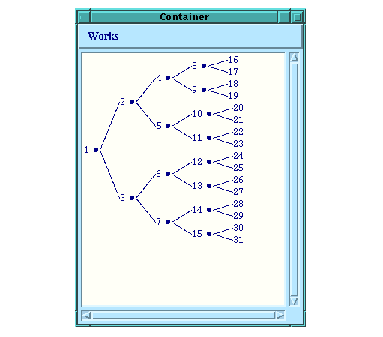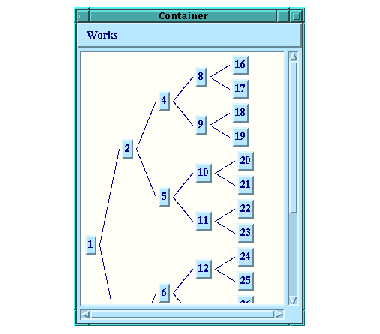### 10.3.1 The implementation of graph panes

One of the major uses the CAPI itself makes of pinboard-objects is to implement graph panes. The ``` graph-pane``` itself is a ``` pinboard-layout``` and it is built using ``` pinboard-object``` s for the nodes and edges. This is because each node (and sometimes each edge) of the graph needs to react individually to the user. For instance, when an event is received by the ``` graph-pane``` , it is told which pinboard object was under the pointer at the time, and it can then use this information to change the selection.

Create the following ``` graph-pane``` and notice that every node in the graph is made from an ``` item-pinboard-object``` as described in the previous section and that each edge is made from a ``` line-pinboard-object``` .

`(defun node-children (node)`
`  (when (< node 16)`
`    (list (* node 2)`
`          (1+ (* node 2)))))`
`(contain`
` (make-instance`
`  'graph-pane`
`  :roots '(1)`
`  :children-function 'node-children)`
` :best-width 300 :best-height 400)`

Figure 10.5 A graph pane with pinboard object nodesAs mentioned before, ``` pinboard-layout``` s can just as easily display ordinary panes inside themselves, and so the ``` graph-pane``` provides the ability to specify the class used to represent the nodes. As an example, here is a ``` graph-pane``` with the nodes made from ``` push-button``` s.

`(contain`
` (make-instance`
`  'graph-pane`
`  :roots '(1)`
`  :children-function 'node-children`
`  :node-pinboard-class 'push-button)`
` :best-width 300 :best-height 400)`

Figure 10.6 A graph pane with push-button nodesLispWorks CAPI User Guide (Unix version) - 8 Apr 2005